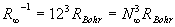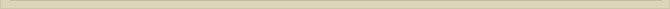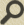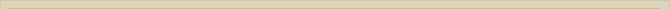## The Quantisation of Distance

When you are interested in physics you must read “Unbelievable“!

Bohr’s Correspondence Principle suggests that equations (5a) and (6) are the same and they appear to be so. Because the energy levels of atoms are discrete, quantified, one can presume that the distance is quantified in some way because the specific energy quantisation of the atom must coincide with certain discrete leaps in orbiting distance. Despite the energy quantification with n  QM classical EM-physics still determines that the increasing quantum level n has to coincide with corresponding increase of orbit distances according to the conservation law of energy.

The equations for the energy level of the atom according to Bohr’s model (6) and the EM-equation (5a) can be seen as identical. We can express the quantum energy levels of the hydrogen atom adequately with: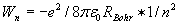(9)

where the radius RBohr is the radius of the hydrogen atom according to Bohr (n=1) and n the principal quantum number.

The distance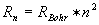of the electron to the nucleus is the macro-world factor that  determines the energy level of the atom and also determines the energy level of Bohr’s atomic model. The quantum number n indicates that for the quantum distances Rn, for n=1,2,3…  the stable ionization energy levels are observed.

Because equation (6) is identical to equation (5a) whenwe derive equation: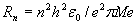(10)

Rn is the orbit distance of the electron in the Bohr-hydrogen atom. The classical Compton-radius Rc of the electron is calculated according to the equation: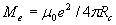Exactly the same radius for the electron is derived in chapter “The Electron” in “From Paradox to Paradigm” where the rest mass/energy of the electron is calculated: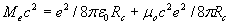where the first part of the equation is the electrostatic energy and the second part the dynamic spin-energy of the electron.

Substitution of Me in (10) and considering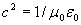we find:

for n=1 the radius of orbit is the Bohr-radius of the hydrogen atom. We calculate the ratio: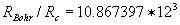The distance ratio between the last energy trap in the atom , the rydberg-distance, and the first, the Bohr-distance, is expressed with ratio (the quantum number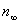):

With equation (8) we calculated that the rydberg principal quantum number is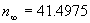. The principal quantum number can also be expressed generally with the ratio:

The difference between (8a) and (8) is that the quantum number N is calculated differently. We will show that the rydberg distance is